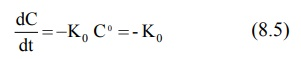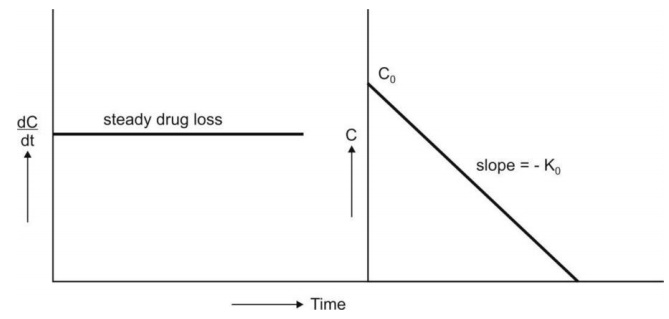# Zero-Order Kinetics (Constant Rate Processes)

| Home | | Biopharmaceutics and Pharmacokinetics |

## Chapter: Biopharmaceutics and Pharmacokinetics : Pharmacokinetics Basic Considerations

the zero-order process can be defined as the one whose rate is independent of the concentration of drug undergoing reaction i.e. the rate of reaction cannot be increased further by increasing the concentration of reactants.

Zero-Order Kinetics (Constant Rate Processes)

If n = 0, equation 8.4 becomes:where Ko = zero-order rate constant (in mg/min)

From equation 8.5, the zero-order process can be defined as the one whose rate is independent of the concentration of drug undergoing reaction i.e. the rate of reaction cannot be increased further by increasing the concentration of reactants.

Rearrangement of equation 8.5 yields:

dC = - K0dt   (8.6)

Integration of equation 8.6 gives:

C – C0 = - K0t       (8.7)

where Co = concentration of drug at t = 0, and

C = concentration of drug yet to undergo reaction at time t.

Equation 8.7 is that of a straight line and states that the concentration of reactant  decreases linearly with time. A plot of C versus t yields such a straight line having slope - Ko and y-intercept Co (Fig.8.2.).Fig. 8.2. Graphs of zero-order kinetics (equations 8.5 and 8.7)